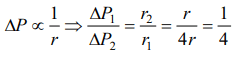## Mechanical Properties of Fluids Questions and Answers Part-12

1. Pressure inside two soap bubbles are 1.01 and 1.02 atmospheres. Ratio between their volumes is
a) 102 : 101
b) $102^{3} : 101^{3}$
c) 8 : 1
d) 2 : 1

Explanation:2. A capillary tube of radius r is dipped in a liquid of density $\rho$ and surface tension S. If the angle of contact is $\theta$, the pressure difference between the two surfaces in the beaker and the capillary
a) $\frac{S}{r}\cos\theta$
b) $\frac{2S}{r}\cos\theta$
c) $\frac{S}{r\cos\theta}$
d) $\frac{2S}{r\cos\theta}$

Explanation:3.The radii of two soap bubbles are $r_{1}$ and $r_{2}$. In isothermal conditions, two meet together in vaccum. Then the radius of the resultant bubble is given by
a) $R=\left(r_{1}+r_{2}\right)\diagup 2$
b) $R=r_{1}\left(r_{1}r_{2}+r_{2}\right)$
c) $R^{2}=r_1^2+r_2^2$
d) $R=r_{1}+r_{2}$

Explanation: $R^{2}=r_1^2+r_2^2$

4. When a large bubble rises from the bottom of a lake to the surface, its radius doubles. If atmospheric pressure is equal to that of column of water height H, then the depth of lake is
a) H
b) 2H
c) 7H
d) 8H

Explanation:5. A soap bubble in vacuum has a radius of 3 cm and another soap bubble in vacuum has a radius of 4 cm. If the two bubbles coalesce under isothermal condition, then the radius of the new bubble is
a) 2.3 cm
b) 4.5 cm
c) 5 cm
d) 7 cm

Explanation:6. The volume of an air bubble becomes three times as it rises from the bottom of a lake to its surface. Assuming atmospheric pressure to be 75 cm of Hg and the density of water to be 1/10 of the density of mercury, the depth of the lake is
a) 5 m
b) 10 m
c) 15 m
d) 20 m

Explanation:7. Excess pressure of one soap bubble is four times more than the other. Then the ratio of volume of first bubble to another one is
a) 1 : 64
b) 1 : 4
c) 64 : 1
d) 1 : 2

Explanation:8. There are two liquid drops of different radii. The excess pressure inside over the outside is
a) More in the big drop
b) More in the small drop
c) Equal in both drops
d) There is no excess pressure inside the drops

Explanation:9. If pressure at half the depth of a lake is equal to 2/3 pressure at the bottom of the lake then what is the depth of the lake
a) 10m
b) 20m
c) 60m
d) 30m